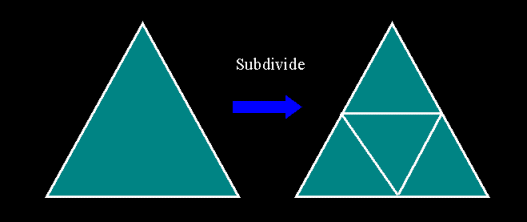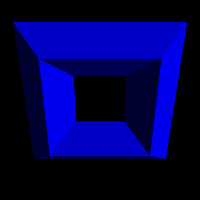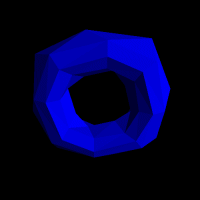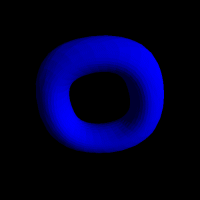Subdivision Surfaces Project Goals The goal of this project was to implement Loop's subdivision surface algorithm, and to provide better insight into the issues associated with implementing the subdivision surfaces in a real-time scene graph. Subdivision Surfaces There are a number of subdivision surface algorithms available: Loop, Modified Butterfly, sqrt(3), Catmull-Clark, etc. There are also a number of extensions to each of these. Some algorithms use triangles, some use polygons. Some are interpolating, and some are approximating (with respect to the control mesh.) They can be uniform or non-uniform. The level of continuity that they deliver to the surface can be different across the algorithms. In the end, the basic algorithm is: Refine the mesh Smooth the mesh Each algorithm has its own definition of how it refines the mesh and what parameters it uses to smooth the mesh. Loop's Subdivision Surface Algorithm Loop's algorithm only works on triangular meshes, which is exactly what we want here. (Triangles are a primitive that is common in our real-time scene graph.) The algorithm is fairly easy to compute and doesn't need to access too many neighboring vertices. (The more neighboring vertices we need, the more we will experience speed and cache issues accessing these additional vertices.) The first step, "refining the mesh" consists of splitting each triangle into 4 sub-triangles. Each edge is split by inserting a new vertex.The position of this new vertex is computed based upon the vertices along the edge and the two neighboring faces. In the following diagram the new vertex is shown in red, and the vertices which will be weighted and combined to generate its position are blue.In the smoothing phase of the algorithm, each vertex from the current level of the mesh is modified with a new position. This new position is calculated based upon a weighting scheme involving its neighboring vertices. The following diagram shows the existing vertex in red and the neighboring vertices which will effect its position in blue.Subdivision ImagesInital Control Mesh: 32 polygons.Subdivision Level 1: 128 polygons.Subdivision Level 2: 512 polygons.Subdivision Level 3: 2048 polygons.Subdivision Level 4: 8192 polygons. All images use facet lighting to highlight the polygons. There are no changes in orientation/zoom between the images, to show the shrinkage caused by an interpolating subdivision surface algorithm. Implementation The implementation of a subdivision surface algorithm requires using data structures which provide connectivity information. The project used a BREP class that was developed in the Progressive Meshing project. Though not specifically designed for this project, where every face is replaced with four other faces each iteration, it was an easy integration. (In future version, I would use a different BREP class or modify the current one to better facilitate the subdivision behavior.) Once the BREP class was added, implementing the algorithm was fairly simple. Tthe process is: split each face, computing new edge vertices, and then modify the position of the pre-existing vertices. When an edge was split, it stored its split vertex index so that the neighboring faces would be able to quickly determine the edge had already been split and which vertex was the newly inserted vertex. Loop's original weighting scheme and Warren/Weimer's less expensive weighting scheme were both investigated. With regard to image quality and surface quality, there was little difference in my tests. Results From the images on the above right, it is easy to see how quickly Loop's algorithm begins to produce smooth surfaces. The images use facet normals to highlight the polygons and by the 4th level of subdivision it is hard to tell. The torus started as a 32 triangle control mesh and the number of triangles quadruples with each subdivision. Performance of the algorithm was quite good until the fourth subdivision. Much of the performance degradation is attributed to the way I had to use the BREP class to implement the algorithm. Future Work Future work for this project is to develop a Subdivision Surface node for the scenegraph using precomputed basis functions to avoid most of the cost of the calculation. The memory requirements for this technique will be investigated. References Loop, Charles, "Smooth Subdivision Surfaces Based On Triangles", Masters Thesis Schroder, Zorin, Siggraph 98 - Subdivision for Modeling and Animation Course Notes Moeller, Haines, Real-time Rendering - 2nd Edition